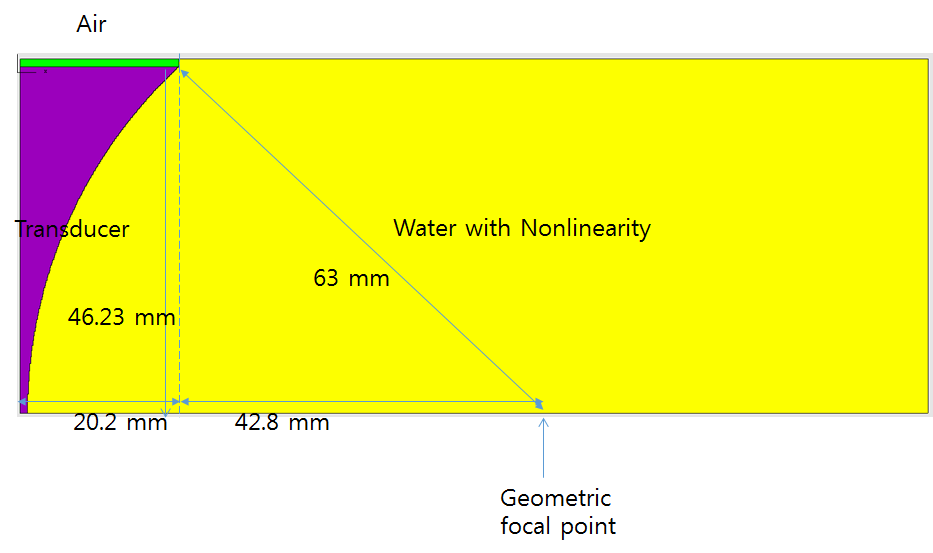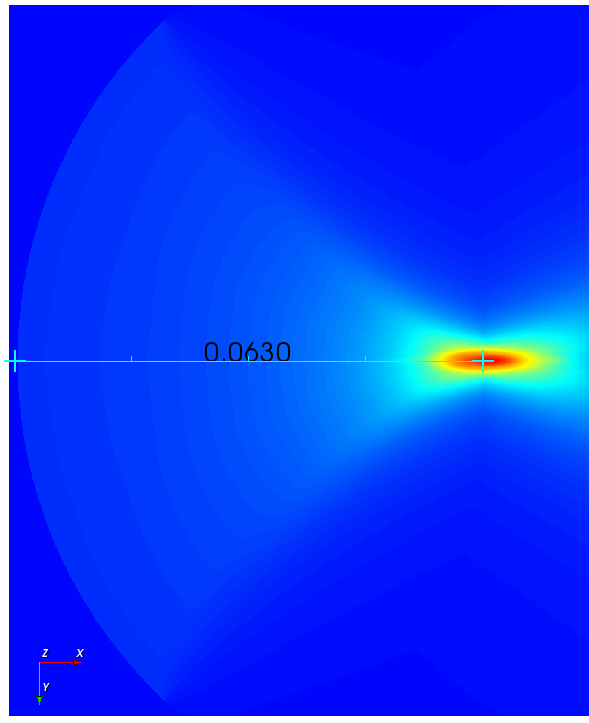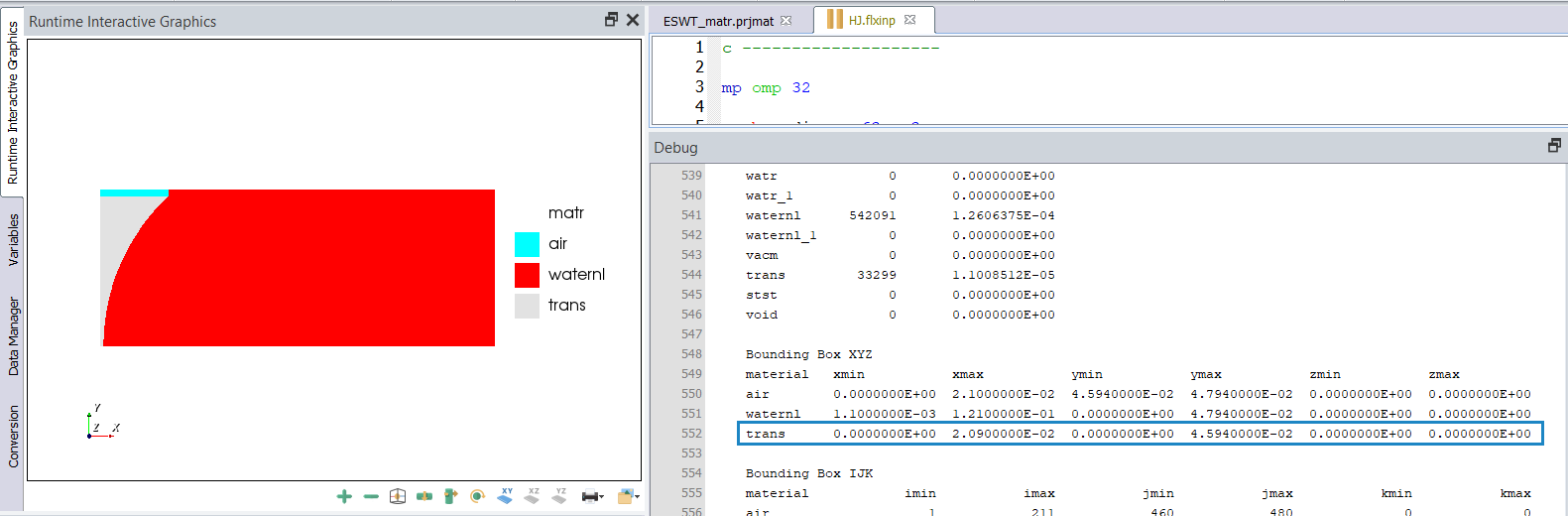# Problem of beam focus point Answered

Hi.

I have problem of beam focus point.

As I ask before, I'm in trouble of prove modeling and result.

I designed concave transducer and fire sine wave, 200kHz, 1 pulse with 1 MPa  (sine 0.2e6 1e6 0 1)Here is my design.

Geometric focal point place 42.8 mm and I expect beam focal point is placed before geometric focal point.

Because in acoustics, beam focal point is formed before geometric focal point.

But result is different.

beam focal point is formed after geometric focal point.

It's not right from acoustics perspective.

I attach my hole code and ask you see this problem.

thanks.

HJ.

--------------------

mp omp 32

symb radius = 63.e-3

symb x1 = 0.0
symb x2 = \$x1 + 21.e-3
symb x3 = \$x2 + 43.11e-3
symb x4 = \$x2 + 100.e-3

symb y1 = 0.0
symb y2 = \$y1 + 45.94e-3
symb y3 = \$y2 + 2.e-3

symb freqint = 0.2e6
symb wavevel = 1500
symb wavemin = \$wavevel / \$freqint
symb nelem = 75
symb box = \$wavemin / \$nelem

symb i1 = 1
symb i2 = \$i1 + nint ( ( \$x2 - \$x1 ) / \$box )
symb i3 = \$i2 + nint ( ( \$x3 - \$x2 ) / \$box )
symb i4 = \$i2 + nint ( ( \$x4 - \$x2 ) / \$box )
symb indgrd = \$i4

symb j1 = 1
symb j2 = \$j1 + nint ( ( \$y2 - \$y1 ) / \$box )
symb j3 = \$j2 + nint ( ( \$y3 - \$y2 ) / \$box )
symb jndgrd = \$j3

grid \$indgrd \$jndgrd axix /* 2D (axisymmetric) (or axiy)

geom
keypnt 4 3
end

symb #read ESWT_matr.prjmat
c -------------------------------------------------------------------------
(this is contents of ESWT_matr.prjmat)
symb epvacm = 8.854e-12 /* dielectric constant for vacumn
symb freqdamp = 1.e6 if noexist /* specified frequency for damping model
symb rmu0 = 1.2566e-6

axis
form vctr
defn posx car2 0. 0. 0. 1. 0. 0. 0. 1. 0.
defn negx car2 0. 0. 0. -1. 0. 0. 0. 1. 0.
defn posy car2 0. 0. 0. 0. 1. 0. 0. 0. 1.
defn negy car2 0. 0. 0. 0. -1. 0. 0. 0. 1.
defn posz car2 0. 0. 0. 0. 0. 1. 1. 0. 0.
defn negz car2 0. 0. 0. 0. 0. -1. 1. 0. 0.
end

matr
c -------------------------------------------------------------------------
c air : Air, room temp
c -------------------------------------------------------------------------
c
type elas
wvsp on
prop air 1.24 343. 0.
elec air \$epvacm
symb eps = \$epvacm * 1.0
elec air \$eps
thrm air 1005. 0.026

c --------------------------------------------------------------
c type : MISC :
c name : vacm :
c desc : Vacuum, also use Void :
c --------------------------------------------------------------

wvsp on
type elas
prop vacm 0 0 0
symb eps = 1
symb aeps = \$epvacm * \$eps
elec vacm \$aeps

c --------------------------------------------------------------
c type : NONLINEAR :
c name : waternl :
c desc : Water - nonlinear for Type TISU :
c --------------------------------------------------------------

wvsp on
type tisu
prop waternl 1000 1500 0 0.1 0.0 0.0
5 -2e+08 2e+08
sdmp waternl \$freqdamp db 0.002 0 1e+06 2 0.01 1
thrm waternl 4178 0.58 0.58 0.58 1.0 0 0 0
symb eps = 1
symb aeps = \$epvacm * \$eps
elec waternl \$aeps

copy waternl waternl_1

end
c -------------------------------------------------------------------------

site
regn air
regn waternl \$i2 \$i4 \$j1 \$j3
regn vacm \$i1 \$i2 \$j1 \$j2
cyln waternl Z 0.0 1.0 \$x3 \$y1 \$radius \$radius 0.0 0.0 0.0 0.0
end

boun
side xmin absr stnd
side xmax absr stnd
side ymin symm all
side ymax absr stnd
end

func sine \$freqint 1e6 0. 1.

calc
pres aprs
max aprs pmin pmax
end

plod
pdef pld1 func /* choose driving function and name 'pld1'
matr mtt1 vacm out
sdf2 pld1 mtt1 vacm waternl /* replace with actual matr names
end

prcs /* run process step

symb #get { step } timestep
symb simtime = 150.e-6 /* Simulation Time
c symb simtime = 3.e-3
symb nexec = nint ( \$simtime / \$step )

symb nloops = 75
c symb nloops = 300
symb nexec_loop = nint ( \$nexec / \$nloops )

grph
set imag avi /* Movie generation
end

proc plot save

exec \$nexec_loop

grph

nvew 4 1
colr tabl data 6 /* Set color table for data
plot 1
mirr y
plot aprs
plot pmax
plot pmin
imag /* Save frame of movie - creates AVI in folder when model executes fully
end
end\$ proc

proc plot \$nloops
stop /* return to command prompt

### 5 comments

• Official comment

Hi there,

I had a look at your model and it seems like the focal point is where it should be according to your diagram.Best Regards,

Chloe

• Hi Chloe

I can get result looks like correct.

But when I find maximum data position, that is not 63 mm.

The maximum value place on 64.5 mm

Is maximum pressure place at 63 mm in data?

• Hi,

The maximum pressure is at 64.7 mm getting the data out of the solver but if you take into account the thickness of the transducer at the beginning then the focal point is less than 63 mm as you expected.

Best Regards,

Chloe

• Hi. Chloe.

That's what I'm asking you.

In Acoustics, beam focus must place in front of geometric focus.

Maximum pressure must place before 63 mm but you said focal point is 63.

You and I did simulation using 'waternl' which have B/A factor.

Then it can't be like that.

I want to know how can I fix it.

Thanks.

• Hi H.J,

If you look at the dimensions of the model in the *.flxprt file, they seem to be different to the ones in your diagram. Transducer length = 20.9 mm, Transducer height = 45.94 mm

(I changed the name of the transducer material to trans so it is easier to see)I would go back and check the geometry so these values match your diagram.

Best Regards,

Chloe

Please sign in to leave a comment.